# NCERT Solutions for Class 9 Maths Exercise 6.2## myCBSEguide App

CBSE, NCERT, JEE Main, NEET-UG, NDA, Exam Papers, Question Bank, NCERT Solutions, Exemplars, Revision Notes, Free Videos, MCQ Tests & more.

NCERT solutions for Class 9 Maths Lines and Angles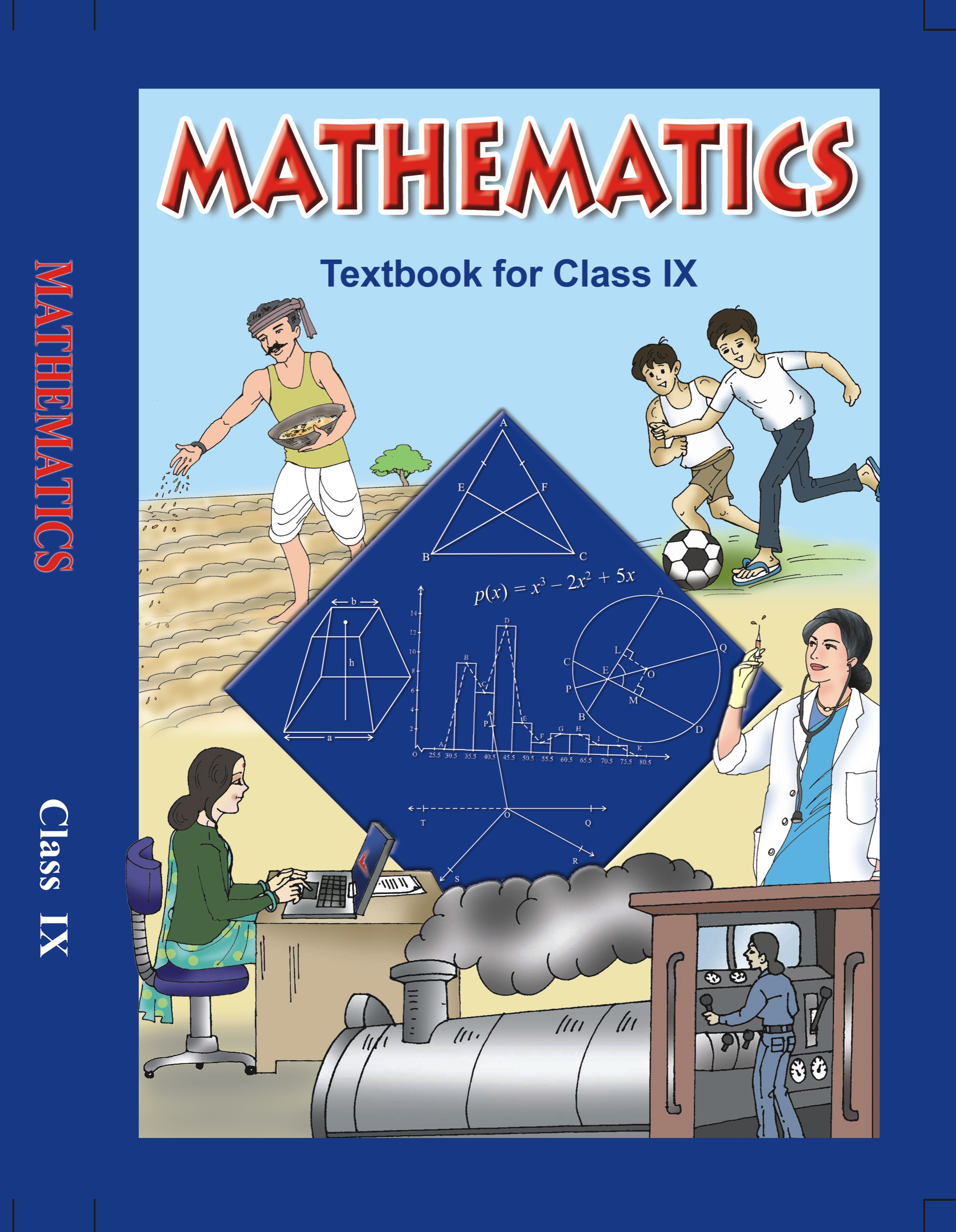## NCERT Solutions for Class 9 Mathematics Lines and Angles

###### 1. In the given figure, find the values of x and y and then show that AB || CD.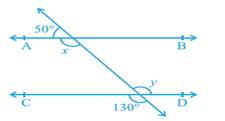Ans. We need to find the value of x and y in the figure given below and then prove that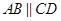.

From the figure, we can conclude that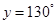(Vertically opposite angles), and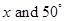form a pair of linear pair.

We know that the sum of linear pair of angles is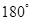.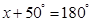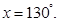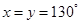.

From the figure, we can conclude that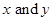form a pair of alternate interior angles corresponding to the lines AB and CD.

Therefore, we can conclude that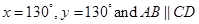.

NCERT Solutions for Class 9 Maths Exercise 6.2

###### 2. In the given figure, if AB || CD, CD || EF and y: z = 3: 7, find x.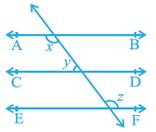Ans. We are given that,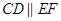and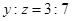.

We need to find the value of x in the figure given below.

We know that lines parallel to the same line are also parallel to each other.

We can conclude that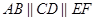.

Let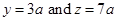.

###### We know that angles on same side of a transversal are supplementary.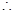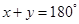.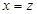(Alternate interior angles)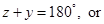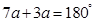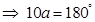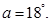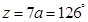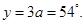Now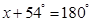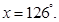Therefore, we can conclude that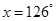.

NCERT Solutions for Class 9 Maths Exercise 6.2

###### 3. In the given figure, If AB || CD,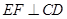and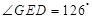, find.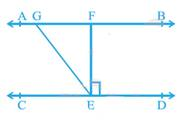Ans. We are given that,and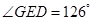.

We need to find the value ofin the figure given below.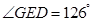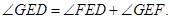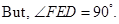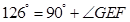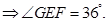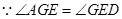(Alternate angles)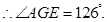From the given figure, we can conclude that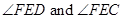form a linear pair.

We know that sum of the angles of a linear pair is.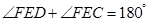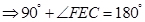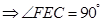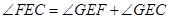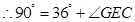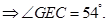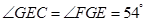(Alternate interior angles)

Therefore, we can conclude that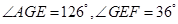and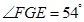.

NCERT Solutions for Class 9 Maths Exercise 6.2

###### 4. In the given figure, if PQ || ST,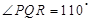and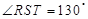, find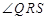.

[Hint: Draw a line parallel to ST through point R.]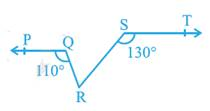Ans. We are given that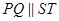,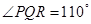and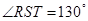.

We need to find the value of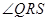in the figure.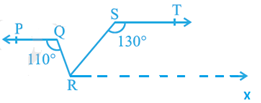We need to draw a line RX that is parallel to the line ST, to get

Thus, we have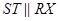.

We know that lines parallel to the same line are also parallel to each other.

We can conclude that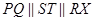.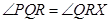, or(Alternate interior angles)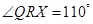.

We know that angles on same side of a transversal are supplementary.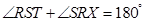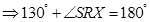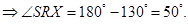From the figure, we can conclude that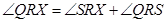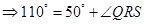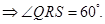Therefore, we can conclude that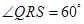.

NCERT Solutions for Class 9 Maths Exercise 6.2

###### 5. In the given figure, if AB || CD,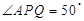and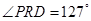, find x and y.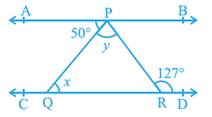Ans. We are given that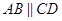,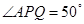and.

We need to find the value of x and y in the figure.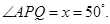(Alternate interior angles)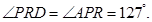(Alternate interior angles)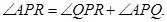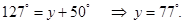Therefore, we can conclude that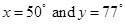.

NCERT Solutions for Class 9 Maths Exercise 6.2

###### 6. In the given figure, PQ and RS are two mirrors placed parallel to each other. An incident ray AB strikes the mirror PQ at B, the reflected ray moves along the path BC and strikes the mirror RS at C and again reflects back along CD. Prove that AB || CD.

Ans. We are given that PQ and RS are two mirrors that are parallel to each other.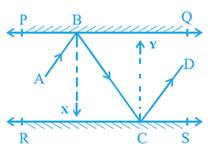We need to prove thatin the figure.

Let us draw lines BX and CY that are parallel to each other, to get

We know that according to the laws of reflection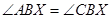and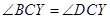.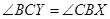(Alternate interior angles)

We can conclude that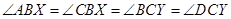.

From the figure, we can conclude that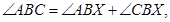and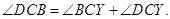Therefore, we can conclude that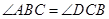.

From the figure, we can conclude that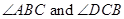form a pair of alternate interior angles corresponding to the lines AB and CD, and transversal BC.

Therefore, we can conclude that## NCERT Solutions for Class 9 Maths Exercise 6.2

NCERT Solutions for Class 9 Maths PDF (Download) Free from myCBSEguide app and myCBSEguide website. Ncert solution class 9 Maths includes text book solutions from Mathematics Book. NCERT Solutions for CBSE Class 9 Maths have total 15 chapters. 9 Maths NCERT Solutions in PDF for free Download on our website. Ncert Maths class 9 solutions PDF and Maths ncert class 9 PDF solutions with latest modifications and as per the latest CBSE syllabus are only available in myCBSEguide.

## CBSE app for Class 9

To download NCERT Solutions for Class 9 Maths, Computer Science, Home Science,Hindi ,English, Social Science do check myCBSEguide app or website. myCBSEguide provides sample papers with solution, test papers for chapter-wise practice, NCERT solutions, NCERT Exemplar solutions, quick revision notes for ready reference, CBSE guess papers and CBSE important question papers. Sample Paper all are made available through the best app for CBSE students and myCBSEguide website.### 8 thoughts on “NCERT Solutions for Class 9 Maths Exercise 6.2”

2. The website is very useful for students like me . Carry on

3. good solitions
thanks for giving this

4. I’m actually going through my yearly CBSE exam of class 9th.I couldn’t understand this exercise in the classroom.So, I was very tensed that how will I do it.But I am thankful to myCBSEguide for helping me with such easy solutions.Once again thanks a lot! I recommend others to go through this…. It’s really helpful.

5. Thanks a lot it is very much helpful

6. It is too gd…whenever I faced prblms in dis exercise…this helped me a lot ?

7. Nice

8. Thanks
I am going to through my half yearly exam .I am not sure about these answers.i was very tensed about what can I do . this help me . thanks a lot again for this